Courses

# Bessel's Special Function (Part - 4) - Mathematical Methods of Physics, UGC - NET Physics Physics Notes | EduRev

## Physics for IIT JAM, UGC - NET, CSIR NET

Created by: Akhilesh Thakur

## Physics : Bessel's Special Function (Part - 4) - Mathematical Methods of Physics, UGC - NET Physics Physics Notes | EduRev

The document Bessel's Special Function (Part - 4) - Mathematical Methods of Physics, UGC - NET Physics Physics Notes | EduRev is a part of the Physics Course Physics for IIT JAM, UGC - NET, CSIR NET.
All you need of Physics at this link: Physics

ASYMPTOTIC EXPANSIONS

Frequently in physical problems there is a need to know how a given Bessel or modiﬁed Bessel function behaves for large values of the argument, that is, the asymptotic behavior.
This is one occasion when computers are not very helpful. One possible approach is to develop a power-series solution of the differential equation, but now using negative powers. This is Stokes’ method. The limitation is that starting from some positive value of the argument (for convergence of the series), we do not know what mixture of solutions or multiple of a given solution we have. The problem is to relate the asymptotic series (useful for large values of the variable) to the power-series or related deﬁnition (useful for small values of the variable). This relationship can be established by introducing a suitable integral representation and then using either the method of steepest descent,  or the direct expansion as developed in this section.

Expansion of an Integral Representation

As a direct approach, consider the integral representation.

1. To show that Kν as given in Eq. (11.122) actually satisﬁes the modiﬁed Bessel equation (11.109).

2. To show that the regular solution Iν is absent.

3. To show that Eq. (11.122) has the proper normalization.

1. The fact that Eq. (11.122) is a solution of the modiﬁed Bessel equation may be veriﬁed by direct substitution. We obtain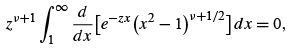which transforms the combined integrand into the derivative of a function that vanishes at both endpoints. Hence the integral is some linear combination of Iν and Kν .

2. The rejection of the possibility that this solution contains Iν constitutes.

3. The normalization may be veriﬁed by showing that, in the limit z → 0,Kν (z) is in agreement with Eq. (11.119). By substituting x = 1 + t/z,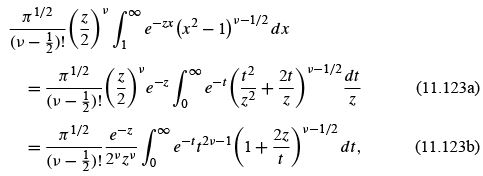taking out t 2 /zas a factor. This substitution has changed the limits of integration to a more convenient range and has isolated the negative exponential dependence e−z . The integral in Eq. (11.123b) may be evaluated for z = 0 to yield (2ν − 1)!. Then, using the duplication formula , we have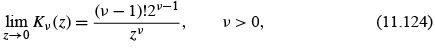in agreement with Eq. (11.119), which thus checks the normalization.23

Now, to develop an asymptotic series for Kν (z), we may rewrite Eq. (11.123a) as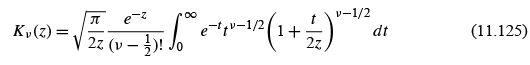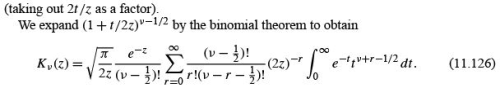Term-by-term integration (valid for asymptotic series) yields the desired asymptotic expansion of Kν (z):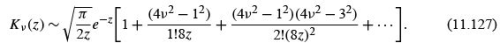Although the integral of Eq. (11.122), integrating along the real axis, was convergent only for −π/2 < arg z< π /2, Eq. (11.127) may be extended to −3π/2 < arg z< 3π/2. Considered as an inﬁnite series, Eq. (11.127) is actually divergent. 24 However, this series is asymptotic, in the sense that for large enough z, Kν (z) may be approximated to any ﬁxed degree of accuracy with a small number of terms.

It is convenient to rewrite Eq. (11.127) as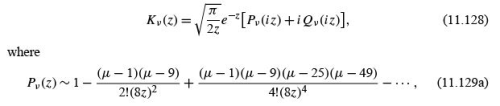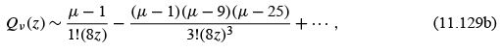and
� = 4ν 2.

It should be noted that although Pν (z) of Eq. (11.129a) and Qν (z) of Eq. (11.129b) have alternating signs, the series for Pν (i z) and Qν (i z) of Eq. (11.128) have all signs positive.
Finally, for z large, Pν dominates.
Then with the asymptotic form of Kν (z), Eq. (11.128), we can obtain expansions for all other Bessel and hyperbolic Bessel functions by deﬁning relations:

1. From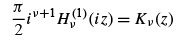(11.130)

we have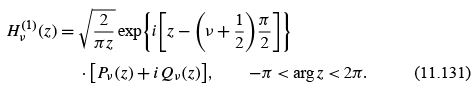2. The second Hankel function is just the complex conjugate of the ﬁrst (for real argument),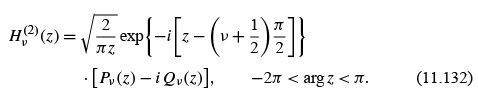An alternate derivation of the asymptotic behavior of the Hankel functions appears in Section 7.3 as an application of the method of steepest descents.

3. Since Jν (z) is the real part of Hν(1) (z) for real z,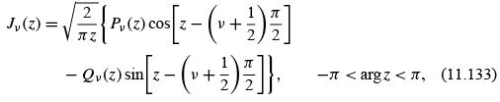holds for real z, that is, arg z = 0,π . Once Eq. (11.133) is established for real z, the relation is valid for complex z in the given range of argument.

4. The Neumann function is the imaginary part of Hν(1) (z) for real z,or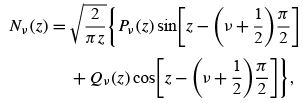−π< arg z< π. (11.134)

Initially, this relation is established for real z, but it may be extended to the complex domain as shown.

5. Finally, the regular hyperbolic or modiﬁed Bessel function Iν (z) is given by

Iν (z) = i −ν Jν (i z)                (11.135)

or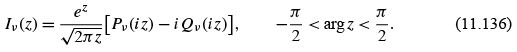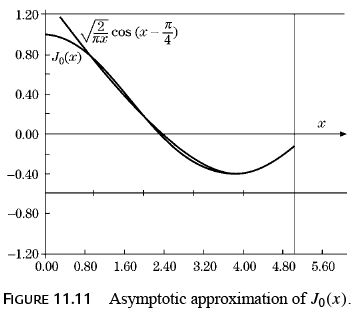This completes our determination of the asymptotic expansions. However, it is perhaps worth noting the primary characteristics. Apart from the ubiquitous z−1/2 , Jν and Nν behave as cosine and sine, respectively. The zeros are almost evenly spaced at intervals of π ; the spacing becomes exactly π in the limit as z →∞. The Hankel functions have been deﬁned to behave like the imaginary exponentials, and the modiﬁed Bessel functions Iν and Kν go into the positive and negative exponentials. This asymptotic behavior may be sufﬁcient to eliminate immediately one of these functions as a solution for a physical problem. We should also note that the asymptotic series Pν (z) and Qν (z), Eqs. (11.129a) and (11.129b), terminate for ν =�1/2, �3/2,... and become polynomials (in negative powers of z). For these special values of ν the asymptotic approximations become exact solutions.
It is of some interest to consider the accuracy of the asymptotic forms, taking just the ﬁrst term, for example (Fig. 11.11),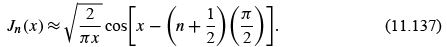Clearly, the condition for the validity of Eq. (11.137) is that the sine term be negligible; that is,

8x ≫ 4n2 − 1.           (11.138)

For n or ν> 1 the asymptotic region may be far out.

As pointed out in Section 11.3, the asymptotic forms may be used to evaluate the various Wronskian formulas .

SPHERICAL BESSEL FUNCTIONS

When the Helmholtz equation is separated in spherical coordinates, the radial equation has the form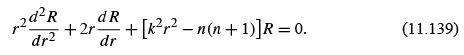This is Eq. (9.65) of Section 9.3. The parameter k enters from the original Helmholtz equation, while n(n + 1) is a separation constant. From the behavior of the polar angle function (Legendre’s equation, Sections 9.5 and 12.5), the separation constant must have this form, with n a nonnegative integer. Equation (11.139) has the virtue of being selfadjoint, but clearly it is not Bessel’s equation. However, if we substitute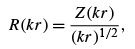Equation (11.139) becomes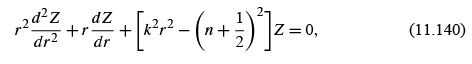which is Bessel’s equation. Z is a Bessel function of order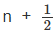(n an integer). Because of the importance of spherical coordinates, this combination, that is,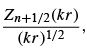occurs quite often.

Deﬁnitions

It is convenient to label these functions spherical Bessel functions with the following deﬁning equations: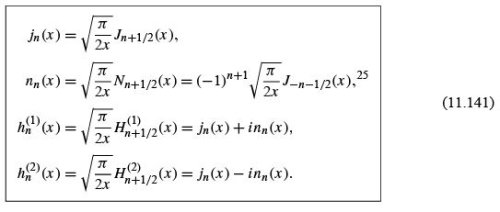These spherical Bessel functions (Figs. 11.13 and 11.14) can be expressed in series form by using the series (Eq. (11.5)) for Jn , replacing n with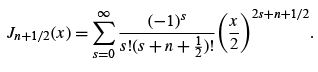(11.142)

Using the Legendre duplication formula,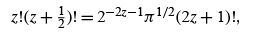(11.143)

we have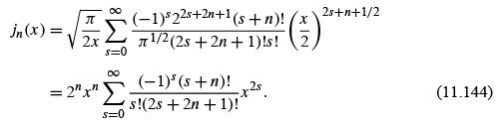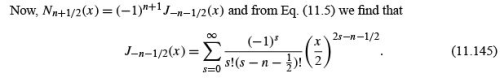This yields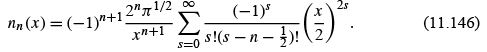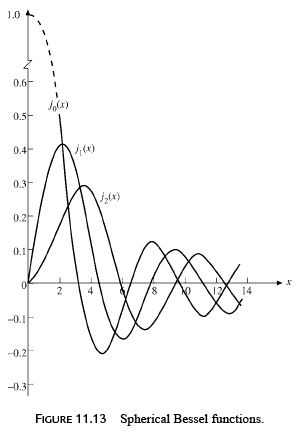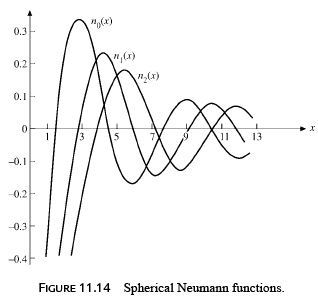The Legendre duplication formula can be used again to give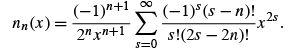(11.147)

These series forms, Eqs. (11.144) and (11.147), are useful in three ways: (1) limiting values as x → 0, (2) closed-form representations for n = 0, and, as an extension of this, (3) an indication that the spherical Bessel functions are closely related to sine and cosine.
For the special case n = 0 we ﬁnd from Eq. (11.144) that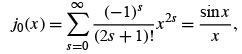(11.148)

whereas for n0 , Eq. (11.147) yields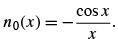(11.149)

From the deﬁnition of the spherical Hankel functions (Eq. (11.141)),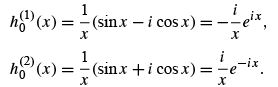(11.150)

Equations (11.148) and (11.149) suggest expressing all spherical Bessel functions as combinations of sine and cosine. The appropriate combinations can be developed from the power-series solutions, Eqs. (11.144) and (11.147), but this approach is awkward. Actually the trigonometric forms are already available as the asymptotic expansion of Section 11.6.
From Eqs. (11.131) and (11.129a),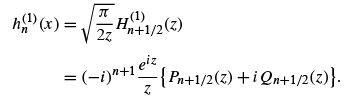(11.151)

Now, Pn+1/2   and Qn+1/2 are polynomials. This means that Eq. (11.151) is mathematically exact, not simply an asymptotic approximation. We obtain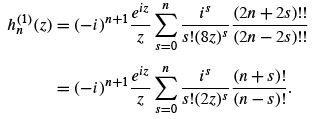(11.152)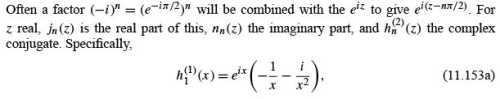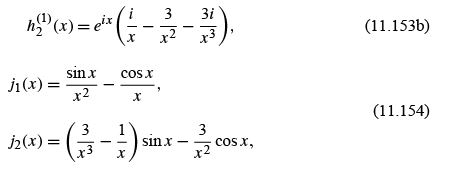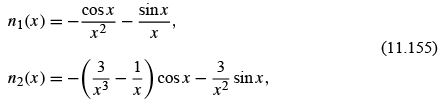and so on.

Limiting Values

For x ≪ 1,26 Eqs. (11.144) and (11.147) yield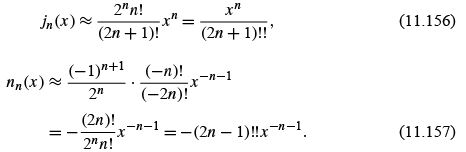The transformation of factorials in the expressions for nn (x ) employs Exercise 8.1.3. The limiting values of the spherical Hankel functions go as �inn (x ).
The asymptotic values of jn ,nn ,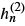, and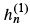may be obtained from the Bessel asymptotic forms, Section 11.6. We ﬁnd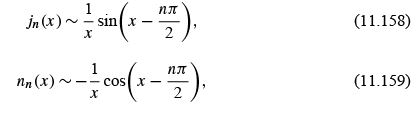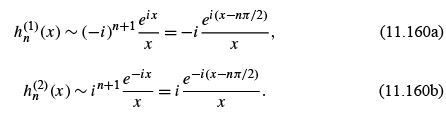The condition for these spherical Bessel forms is that x ≫ n(n + 1)/2. From these asymptotic values we see that jn (x ) and nn (x ) are appropriate for a description of standing spherical waves;(x ) and(x ) correspond to traveling spherical waves. If the time dependence for the traveling waves is taken to be e−iωt , then(x ) yields an outgoing traveling spherical wave,(x ) an incoming wave. Radiation theory in electromagnetism and scattering theory in quantum mechanics provide many applications.

Recurrence Relations

The recurrence relations to which we now turn provide a convenient way of developing the higher-order spherical Bessel functions. These recurrence relations may be derived from the series, but, as with the modiﬁed Bessel functions, it is easier to substitute into the known recurrence relations (Eqs. (11.10) and (11.12)). This gives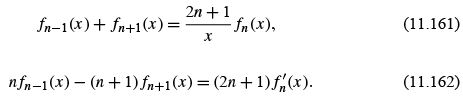Rearranging these relations (or substituting into Eqs. (11.15) and (11.17)), we obtain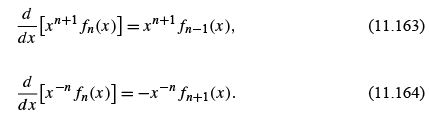Here fmay represent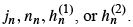The speciﬁc forms, Eqs. (11.154) and (11.155), may also be readily obtained from Eq. (11.164).
By mathematical induction we may establish the Rayleigh formulas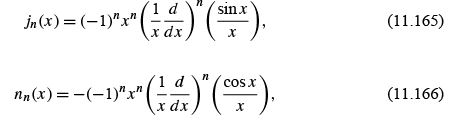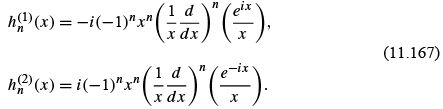Orthogonality

We may take the orthogonality integral for the ordinary Bessel functions (Eqs. (11.49) and (11.50)),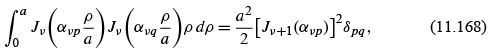and substitute in the expression for jn to obtain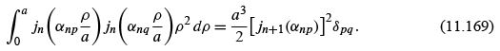Here αnp and αnq are roots of jn .
This represents orthogonality with respect to the roots of the Bessel functions. An illustration of this sort of orthogonality is provided in Example 11.7.1, the problem of a particle in a sphere. Equation (11.169) guarantees orthogonality of the wave functions jn (r ) for ﬁxed n. (If n varies, the accompanying spherical harmonic will provide orthogonality.)

Example : PARTICLE IN A SPHERE

An illustration of the use of the spherical Bessel functions is provided by the problem of a quantum mechanical particle in a sphere of radius a . Quantum theory requires that the wave function ψ , describing our particle, satisfy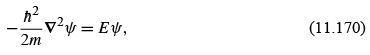and the boundary conditions (1) ψ(r ≤ a) remains ﬁnite, (2) ψ(a ) = 0. This corresponds to a square-well potential V = 0, r ≤ a , and V =∞, r> a .Here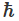is Planck’s constant divided by 2π, m is the mass of our particle, and E is, its energy. Let us determine the minimum value of the energy for which our wave equation has an acceptable solution.
Equation (11.170) is Helmholtz’s equation with a radial part :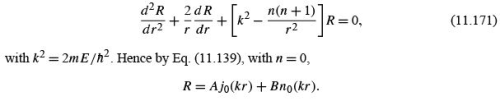We choose the orbital angular momentum index n = 0, for any angular dependence would raise the energy. The spherical Neumann function is rejected because of its divergent behavior at the origin. To satisfy the second boundary condition (for all angles), we require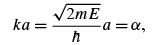(11.172)

where α is a root of j, that is, j0 (α) = 0. This has the effect of limiting the allowable energies to a certain discrete set, or, in other words, application of boundary condition (2) quantizes the energy E . The smallest α is the ﬁrst zero of j0 ,

α = π,

and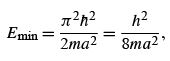(11.173)

which means that for any ﬁnite sphere the particle energy will have a positive minimum or zero-point energy. This is an illustration of the Heisenberg uncertainty principle for Δp with Δr ≤ a .
In solid-state physics, astrophysics, and other areas of physics, we may wish to know how many different solutions (energy states) correspond to energies less than or equal to some ﬁxed energy E0 . For a cubic volume  the problem is fairly simple.

Another form, orthogonality with respect to the indices, may be written as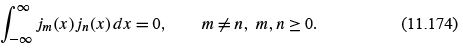The proof is left as Exercise 11.7.10. If m = n (compare Exercise 11.7.11), we have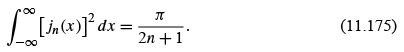Most physical applications of orthogonal Bessel and spherical Bessel functions involve orthogonality with varying roots and an interval [0,a ] and Eqs. (11.168) and (11.169) for continuous-energy eigenvalues.
The spherical Bessel functions will enter again in connection with spherical waves, but further consideration is postponed until the corresponding angular functions, the Legendre functions, have been introduced.

Offer running on EduRev: Apply code STAYHOME200 to get INR 200 off on our premium plan EduRev Infinity!

159 docs

,

,

,

,

,

,

,

,

,

,

,

,

,

,

,

,

,

,

,

,

,

,

,

,

;